##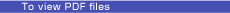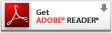Feature Articles: Communication Science for Achieving Coexistence and Co-creation between Humans and AI

# Transmission of Messages to the Efficiency Limit—Implementation of Tractable Channel Code Achieving the Shannon Limit

## Abstract

This article introduces CoCoNuTS, a technology for implementing an error correcting code (channel code) that achieves the efficient transmission limit known as the Shannon limit. It was once believed that a huge time complexity was necessary to achieve the Shannon limit for a given channel. However, practical channel codes that achieve the Shannon limit have recently been developed, but these codes achieve the Shannon limit only for a restricted class of channels. We have proven mathematically that we can construct codes achieving the Shannon limit with our CoCoNuTS technology. Furthermore, we have confirmed experimentally that the implemented codes outperform conventional codes for a channel where it is impossible to achieve the Shannon limit using the conventional codes.

Keywords: information theory, channel code, CoCoNuTS

## 1. Introduction

To achieve effective communication, messages must be transmitted correctly over noisy environments. Channel codes, also known as error correcting codes, represent technology that provides error-free transmission. They are applied to transmissions over optical fiber and radio environments as well as to computers, recorders such as hard/optical discs, and two-dimensional codes. It is not too much to say that they are implemented in almost all communication devices.

For a given noisy environment (channel), there is a transmission efficiency limit to achieve error-free transmission. This is called the Shannon limit*1 after the computer scientist C. E. Shannon, who presented this limit in 1948. However, his code construction is impractical in the sense that it requires a huge amount of time. The information theory community has been studying construction of practical codes designed to achieve the Shannon limit for around 70 years.

Low-density parity check (LDPC) codes*2 and polar codes have recently been developed as practical codes that achieve the Shannon limit. They are implemented in fifth-generation (5G) mobile communication technology. However, their codes do not achieve the Shannon limit for all channels but only for a particular class.

 *1 The Shannon limit: A generic name representing the fundamental performance limit of transmission (source codes, channel codes, and codes for information-theoretic security) derived by information theory. The name refers to the computer scientist C. E. Shannon, who was the founder of information theory. In the context of a channel code (error correcting code), this limit is also called the channel capacity, where higher speed transmission is possible when the limit is increased. When the efficiency of the codes reaches the limit by increasing the number of transmitted signals, we say that these codes have achieved the Shannon limit. *2 LDPC codes: A class of codes that are tractable and that achieve the Shannon limit for a particular class of channels. A sparse matrix (where almost all the elements are zero) is used for practical decoding. It was introduced by computer scientist R. G. Gallager in 1962 but was not practically implemented because of the limited computer power at that time. It was re-evaluated in the 1990s and is implemented in wireless local area networks, satellite digital broadcasting, and 5G mobile communication technology.

## 2. Research accomplishment

CoCoNuTS*3, a coding technology that achieves the Shannon limit, was developed at NTT Communication Science Laboratories. We can apply this technology to construct channel codes as well as source codes and codes for information-theoretic security that are tractable and achieve the fundamental limit of transmission. In this article, we describe how we applied this technology to channel codes and proved mathematically that we can use them to achieve the Shannon limit [1–3]. Furthermore, we confirmed experimentally that our codes outperform LDPC codes in a channel where the Shannon limit is not achievable with LDPC codes.

 *3 CoCoNuTS: The name of a technology developed by NTT to design codes that achieve the Shannon limit. We call this technology CoCoNuTS (Code based on Constrained Numbers Theoretically achieving the Shannon limit) because it uses constrained-random-number generators to achieve the fundamental limit.

## 3. Coding technology

In this section, we briefly describe channel coding. We also explain the Shannon limit and introduce our newly developed CoCoNuTS technology.

### 3.1 Communication system achieved using channel codes

A communication system achieved using channel codes is shown in Fig. 1. In this figure, the sender is a wireless station that is sending messages, and the receiver is a user who has a smartphone. The encoder converts a message M to a signal X called a channel input. It is transmitted as a modulated radio wave, where it is assumed that noise is added to the transmission. The decoder converts a reproduction M’ from a received signal Y called a channel output. The transmission is successful when M = M’ is satisfied, and the decoding error probability is defined as the probability of events satisfying MM’.Fig. 1. Communication system realized using channel codes.

The transmission efficiency, hereafter called the coding rate, is defined as the number of message symbols divided by the number of transmitted signals. A higher coding rate means higher speed transmission. However, a coding rate that is too high makes it impossible to achieve a decoding error probability close to zero. Our goal is to construct an encoder and decoder pair where the decoding error probability is close to zero and the coding rate is close to the fundamental limit.

### 3.2 Example of channel coding

An example of channel coding of the message “01” is shown in Fig. 2. The encoder converts the message to the channel input signal “01011,” which is a twofold repetition of the message and a 1-bit parity check, which is the sum (exclusive-or) of two message bits. This operation is called encoding. In this example, the decoder observes the channel output signal “01111.” The decoder determines the position of the noise by using the encoding rule and reproduces the original message. This operation is called decoding. In this example, the message is successfully reproduced, but a noisier output may prevent us from reproducing the original message. In Fig. 2, a 2-bit message is encoded in a 5-bit channel input, where the coding rate is 2/5 = 0.4. By increasing the number of repetitions and parity check bits, the number of noisy bits that the decoder can specify is increased while the coding rate is decreased. For high-speed error-free transmission, it is necessary to specify all noisy bits with a probability close to one and obtain the highest possible encoding rate.Fig. 2. Example of channel coding.

### 3.3 Shannon limit

The Shannon limit (channel capacity) is defined in Fig. 3. As shown in Fig. 1, the decoding error probability of a code must be close to zero. However, a more efficient code has a higher coding rate. The Shannon limit is the optimum coding rate of codes, where the decoding error probability is close to zero. Shannon derived the limit illustrated in Fig. 3, where the optimum is achievable by letting the number of transmitted signals reach infinity. It is theoretically impossible to construct codes with a rate beyond the Shannon limit. It should be noted that we have to optimize the channel input distribution P, which appears in the maximum operator on the right-hand side of the equality.Fig. 3. The Shannon limit.

### 3.4 Proposed method CoCoNuTS

The construction of a channel code using CoCoNuTS is shown in Fig. 4. With the proposed method, a code is constructed by using two sparse matrices (almost all elements of a matrix are zero) A and B, and a vector c. Functions fA,B and gA (red squares in Fig. 4) are implemented by using tractable constrained-random-number generators [1–3]. We can achieve the Shannon limit by employing an optimal channel input distribution P.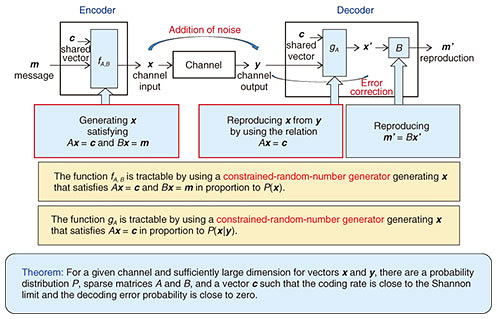Fig. 4. Proposed method CoCoNuTS.

In contrast, a generator matrix is used as the encoder of the conventional LDPC codes, where the channel input distribution is approximately uniform. This implies that the LDPC codes achieve the Shannon limit if the maximum in Fig. 3 is achieved with a uniform input distribution P, and otherwise they cannot achieve the limit.

### 3.5 Experimental results

In Fig. 5, the proposed codes are compared with the conventional LDPC codes. The horizontal axis represents the coding rate, where a larger value implies better performance. The vertical axis represents the decoding error probability, where a smaller value implies better performance. The graph shows that the proposed codes outperform the LDPC codes. For example, when they are compared on the horizontal line at a decoding error probability of 10−4, the proposed codes can transmit 60 bits of messages more than the LDPC codes per 2000 bits of transmitted signals.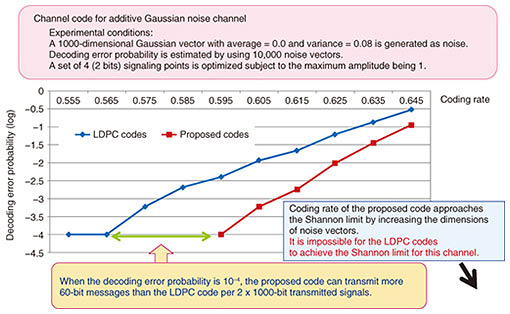Fig. 5. Experimental results.

A comparison at the same coding rate of 0.6 is shown in Fig. 6. The black dots represent the decoding error events, where encoding and decoding are repeated 1200 times under the conditions described in Fig. 5. There is no decoding error event with the proposed code, while there are seven decoding error events with the LDPC code. This result suggests that the proposed code is more reliable than the conventional code in the same noisy environment and with the same coding rate.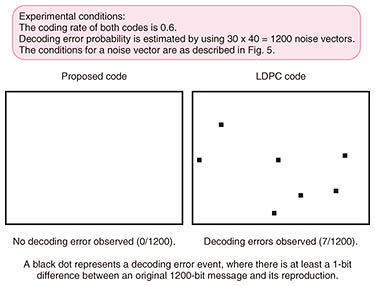Fig. 6. Visualization of decoding error events at a coding rate of 0.6.

A comparison when a compressed image file (JPEG)*4 is transmitted, where it is assumed that there is no decoding error, is shown in Fig. 7. In this situation, a decoding error destroys the file, and most of the original image cannot be reproduced. When the coding rate is 0.5, both the proposed code and the LDPC code can reproduce the original image. However, the LDPC code cannot reproduce the original image at a coding rate of 0.6. This implies that the coding rate limit of the LDPC code is between 0.5 and 0.6, which is less than that of the proposed code.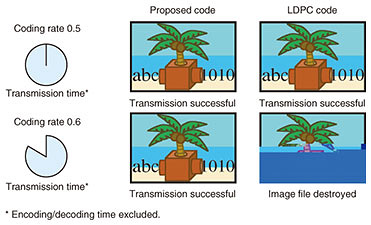Fig. 7. Transmission of compressed image at a coding rate of 0.6.

 *4 JPEG: JPEG is a standard format for image compression developed by the Joint Photographic Experts Group.

## 4. Future work

Our goal is to achieve future high-speed digital communication by establishing related peripheral technologies. We will continue our research in this area and report our results.

## References

 J. Muramatsu and S. Miyake, “Concept of CoCoNuTS,” Proc. of the 10th Asia-Europe Workshop on Information Theory, p. 4, Boppard, Germany, June 2017. J. Muramatsu, “Channel Coding and Lossy Source Coding Using a Constrained Random Number Generator,” IEEE Trans. Inf. Theory, Vol. 60, No. 5, pp. 2667–2686, May 2014. J. Muramatsu and S. Miyake, “Channel Code Using Constrained-random-number Generator Revisited,” IEEE Trans. Inf. Theory, Vol. IT-65, No. 1, pp. 500–508, Jan. 2019.Jun Muramatsu Research Scientist, NTT Communication Science Laboratories. He received a B.S. and M.S. in mathematics and a Ph.D. from Nagoya University, Aichi, in 1990, 1992, and 1998. He joined NTT Transmission Systems Laboratories in 1992 and moved to NTT Communication Science Laboratories in 1995. He has been conducting research on information theory. From February 2007 to February 2008, he was a visiting researcher at ETH Zurich, Switzerland. From 2006 to 2010, he was an associate editor of the Institute of Electronics, Information and Communication Engineers (IEICE) Transactions on Fundamentals of Electronics, Communications and Computer Sciences. He has been Chair of the IEICE Technical Committee on Information Theory since 2018. He received the Young Researcher Award from SITA (the Society of Information Theory and Its Application) in 2003 and the 63rd Best Paper Award from IEICE in 2007. He is a member of IEICE and the IEEE (Institute of Electrical and Electronics Engineers) Information Theory Society.

↑ TOP